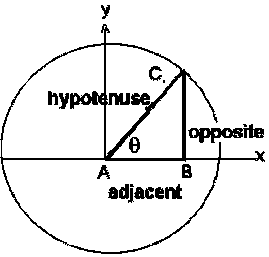# Trigonometry Calculator

The trigonometric functions have been defined using the unit circle.

 Sin Cos Tan Cot Sec Cosec
 Arc Sin Arc Cos Arc Tan Arc Cot Arc Sec Arc Cosec

### Degrees

 Sin Cos Tan Cot Sec Cosec
 Arc Sin Arc Cos Arc Tan Arc Cot Arc Sec Arc Cosec

The sin(θ) is the vertical component , the cos(θ) is the horizontal coordinate of the arc endpoint.

where

Sin(q) = Opposite / HypotenuseThe table for basic trigonometric numbers of the most common angles as follows:

Angle  sin  cos  tan  cot  sec  cosec
0o 0 1 0 Not define 1 Not define
30o 1/2 √3/2 1/√3 √3 2/√3 2
45o 1/√2 1/√2 1 1 √2 √2
60o √3/2 1/2 √3 1/√3 2 2/√3
90o 1 0 Not define 0 Not define 1

### Difference between radian (RED) and degrees (DEG)

A radian is equal to 180 degrees because a whole circle is 360 degrees and is equal to two pi radians. A radian is not as widely used in the measurement of circles and angles as a degree because it involves the knowledge of higher mathematics and includes tangents, sine, and cosines. A sin(θ) is the vertical component and cos(θ) is the horizontal coordinate of the arc endpoint.